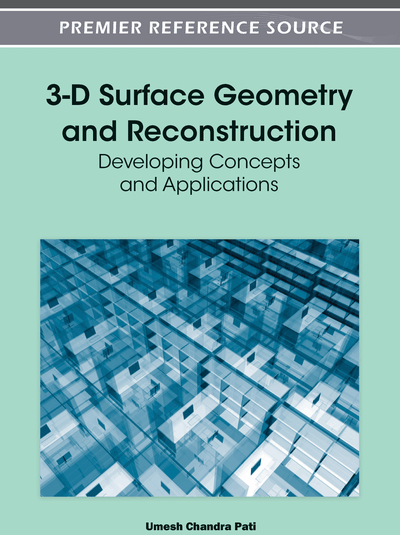# Surface Modelling Using Discrete Basis Functions for Real-Time Automatic Inspection

Paul O’Leary (Institute for Automation, University of Leoben, Austria) and Matthew Harker (Institute for Automation, University of Leoben, Austria)
DOI: 10.4018/978-1-4666-0113-0.ch010

## Abstract

This chapter presents an introduction to discrete basis functions and their application to real-time automatic surface inspection. In particular, discrete polynomial basis functions are analyzed in detail. Emphasis is placed on a formal and stringent mathematical background, which enables an analytical a-priori estimation of the performance of the methods for specific applications. A generalized synthesis algorithm for discrete polynomial basis functions is presented. Additionally a completely new approach to synthesizing constrained basis functions is presented. The resulting constrained basis functions form a unitary matrix, i.e. are optimal with respect to numerical error propagation and have many applications, e.g. as admissible functions in Galerkin methods for to solution of boundary value and initial value problems. Furthermore, a number of case studies are presented, which show the applicability of the methods in real applications.
Chapter Preview
Top

## 1.1 Surface Measurement

The methods presented in this chapter are generally applicable to any data which lies on a regular grid, more precisely called an invariant lattice (see Figure 1). The term regular or invariant refers to the fact that there is a constant number of data points in each of the two dimensions of the grid; it is not necessary that the points be equally spaced. In the case of image processing we will most commonly be dealing with grids with constant spacing in the x and y directions, simply because of the structure of the pixels in a camera chip. However, polynomially distorted grids, see Figure 1c, are encountered in non-rigid registration. Also conformal mappings such as polar coordinates can be dealt with using the methods presented here.

Figure 1.

Some examples of invariant grids: a) an invariant grid with equal spacing in the x and y directions; b) exponential spacing in the x and y directions; c) a polynomially distorted grid; d) polar grid, here the terms x and y directions are not appropriate

In this chapter we work primarily with data acquired using a laser scanning device. The device uses a plane of light, also called light sectioning, to acquire cross sectional profiles. This, combined with motion, enables the acquisition of complete surfaces. The data acquired in this manner have in general different resolutions in the x and y directions. The resolution along the laser line is determined by the camera, whereby the resolution in the other direction is determined by the rate at which the sections can be measured and the speed of the material being observed.

## Complete Chapter List

Search this Book:
Reset